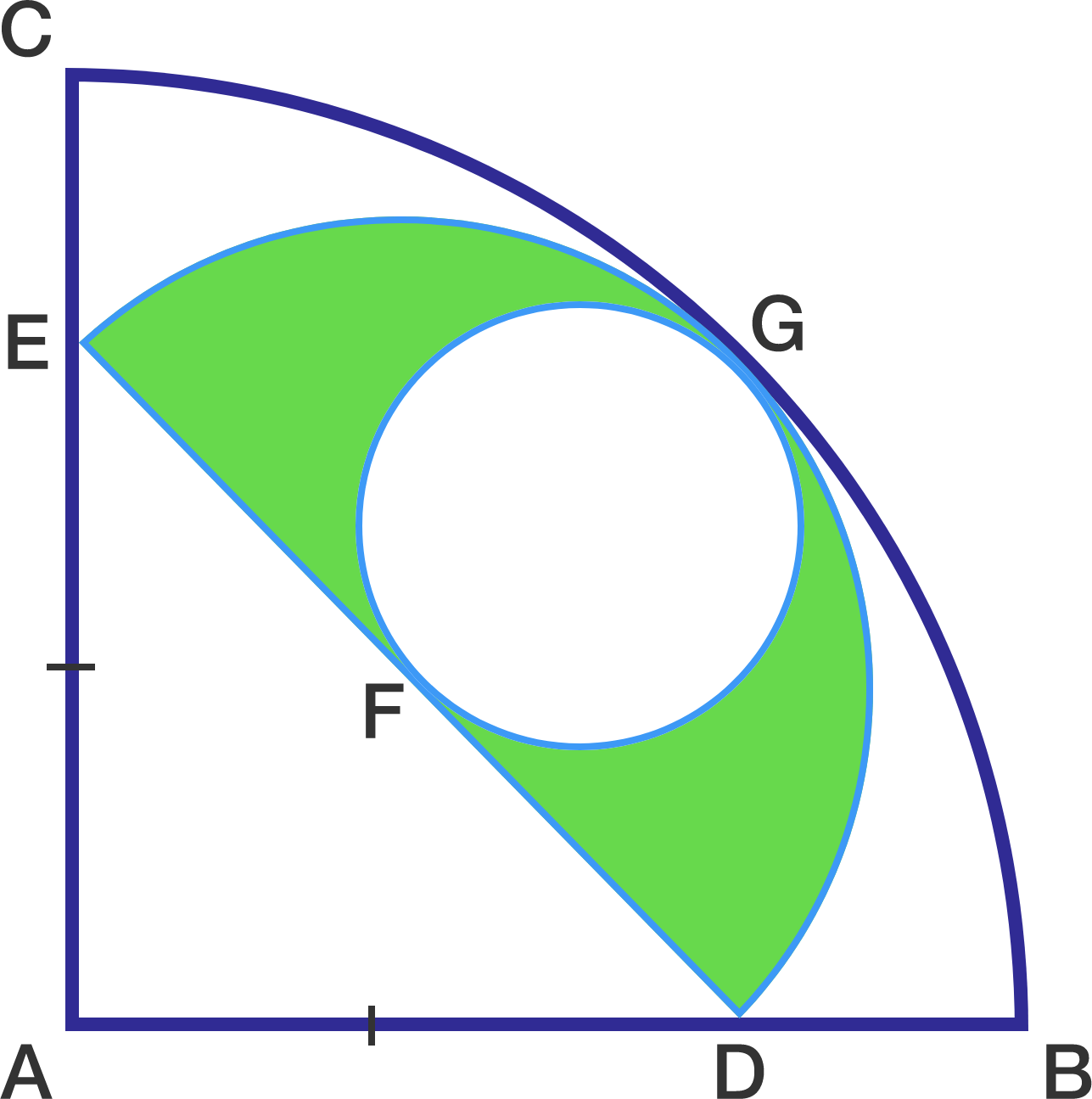# Circle In A Semicircle In A Quarter Circle

Geometry Level 2The diagram above shows that a semicircle is inscribed in a quarter circle while a small circle is inscribed in the semicircle. Given that $AD=AE$ and the radius of the quarter circle is $14\sqrt{2} \text{ cm}$, find the area of the green region above (in $\text{cm}^{2}$).

For your final step, use the approximation $\pi = \dfrac{22}{7}$.

×

Problem Loading...

Note Loading...

Set Loading...